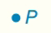Chapter 7.CT, Problem 4CTElementary Geometry For College St...

7th Edition
Alexander + 2 others
ISBN: 9781337614085

Solutions

Chapter
SectionElementary Geometry For College St...

7th Edition
Alexander + 2 others
ISBN: 9781337614085
Textbook Problem

Describe the locus of points in a plane that are at a distance of 3 cm from point P.Exercises 4, 5

To determine

To describe:

The locus of points in a plane that are at a distance of 3 cm from point P.

Explanation

Definition:

Locus of points that are at a fixed distance r from fixed point P.

For example:

The circle with center P and radius r.

Calculation:

A locus is always an equal distance away from the given point...

Still sussing out bartleby?

Check out a sample textbook solution.

See a sample solution

The Solution to Your Study Problems

Bartleby provides explanations to thousands of textbook problems written by our experts, many with advanced degrees!

Get Started

In Problems 25-30, suppose that 25. What is ?

Mathematical Applications for the Management, Life, and Social Sciences

In Exercises 5-8, find the limit. limx01x(x+x)

Calculus: An Applied Approach (MindTap Course List)

The length of the curve given by x = 3t2 + 2, y = 2t3, is:

Study Guide for Stewart's Multivariable Calculus, 8th

True or False: If f(x) = f (−x) for all x then .

Study Guide for Stewart's Single Variable Calculus: Early Transcendentals, 8th